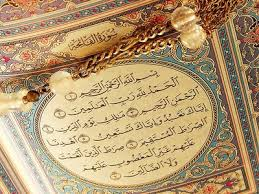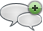### A note about 7^n + 29^n + 139^n

#### الجمعة، 17 إبريل 2015 - 1:50(Regarding the numbers in Surat Alfateha:  7 ayats, 29 words, 139 letters)

Theorem:

7n + 29n + 139n is always a composite number for every n. Proof. Note that 29 + 139 = 168 = 7 × 24, hence for odd number n, 29n + 139n has a factor 29 + 139. Thus the result holds. So we assume n = 2k.Let

φ(k) = 72k + 292k + 1392k = 49k + 841k + 19321k .

Note that

49 ≡ 1(mod 3),

841 ≡ 1(mod 3),

19321 ≡ 1(mod 3),

then

49k ≡ 1(mod 3),

841k ≡ 1(mod 3),

19321k ≡ 1(mod 3).

Therefore, 49k + 841k + 19321k ≡ 3(mod 3), that is 3|49k + 841k + 19321k .أضف تعليقك
##### رجاء ضرورة الإلتزام بعدم إضافة أي تعليق يمس أو يسيء للأديان أو المعتقدات أو المقدسات, كما نرجو ألا يتضمن التعليق السباب أو أي ألفاظ تخدش الحياء والذوق العام

 الأكثر قراءةالسجدة.ملاحظة في ترتيب سور فصلت والشورى وق.Suras Whose Number and Number of Ayas are Both Prime.تعيين ليلة القدر من سورة الدخان.تعيين ليلة القدر.Special numbers of Quran.الكهف.Number 114 is an Indication of Completeness.مزيد من الاثبات ان عاد الثانية هي امريكا.Interesting Fact About Sura Nooh 71.
الموقع الرسمي حم © 2015, كل الحقوق محفوظة |